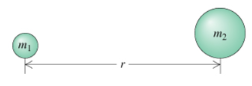# Problem: Consider the earth following its nearly circular orbit (dashed curve) about the sun. The earth has mass 5.98 x 1024 kg and the sun has mass  1.99 x 1030 kg. They are separated, center to center, by r = 93 million miles = 150 million km. What is the magnitude of the gravitational force acting on the earth due to the sun?

###### FREE Expert Solution

The gravitational force is:

$\overline{){{\mathbf{F}}}_{{\mathbf{g}}}{\mathbf{=}}\frac{\mathbf{G}{\mathbf{m}}_{\mathbf{1}}{\mathbf{m}}_{\mathbf{2}}}{{\mathbf{r}}^{\mathbf{2}}}}$

89% (435 ratings)###### Problem Details

Consider the earth following its nearly circular orbit (dashed curve) about the sun.The earth has mass 5.98 x 1024 kg and the sun has mass  1.99 x 1030 kg. They are separated, center to center, by r = 93 million miles = 150 million km.

What is the magnitude of the gravitational force acting on the earth due to the sun?